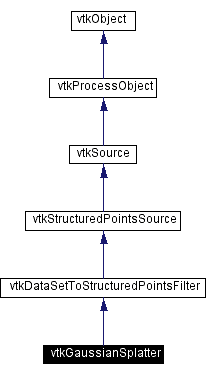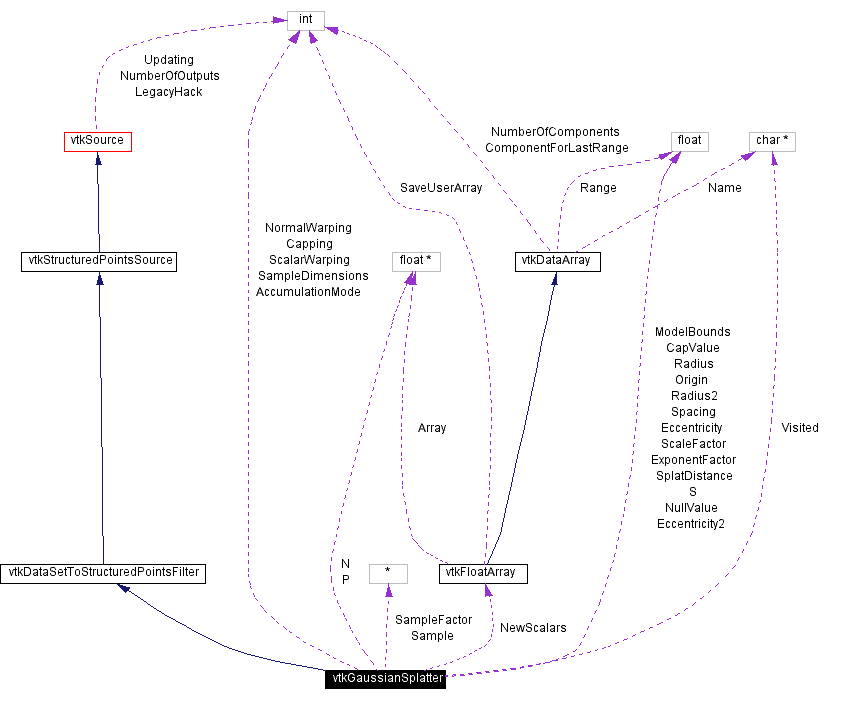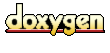Main Page   Class Hierarchy   Alphabetical List   Compound List   File List   Compound Members   File Members   Related Pages

# vtkGaussianSplatter Class Reference

splat points into a volume with an elliptical, Gaussian distribution. More...

`#include <vtkGaussianSplatter.h>`

Inheritance diagram for vtkGaussianSplatter:[legend]
Collaboration diagram for vtkGaussianSplatter:[legend]
List of all members.

## Public Methods

virtual const char * GetClassName ()
virtual int IsA (const char *type)
void PrintSelf (ostream &os, vtkIndent indent)
void ComputeModelBounds ()
void SetSampleDimensions (int i, int j, int k)
void SetSampleDimensions (int dim)
virtual int * GetSampleDimensions ()
virtual void GetSampleDimensions (int data)
virtual void SetModelBounds (float, float, float, float, float, float)
virtual void SetModelBounds (float)
virtual float * GetModelBounds ()
virtual void GetModelBounds (float data)
virtual void SetScaleFactor (float)
virtual float GetScaleFactor ()
virtual void SetExponentFactor (float)
virtual float GetExponentFactor ()
virtual void SetNormalWarping (int)
virtual int GetNormalWarping ()
virtual void NormalWarpingOn ()
virtual void NormalWarpingOff ()
virtual void SetEccentricity (float)
virtual float GetEccentricity ()
virtual void SetScalarWarping (int)
virtual int GetScalarWarping ()
virtual void ScalarWarpingOn ()
virtual void ScalarWarpingOff ()
virtual void SetCapping (int)
virtual int GetCapping ()
virtual void CappingOn ()
virtual void CappingOff ()
virtual void SetCapValue (float)
virtual float GetCapValue ()
virtual void SetAccumulationMode (int)
virtual int GetAccumulationMode ()
void SetAccumulationModeToMin ()
void SetAccumulationModeToMax ()
void SetAccumulationModeToSum ()
const char * GetAccumulationModeAsString ()
virtual void SetNullValue (float)
virtual float GetNullValue ()

## Static Public Methods

int IsTypeOf (const char *type)
vtkGaussianSplatter * SafeDownCast (vtkObject *o)
vtkGaussianSplatter * New ()

## Protected Methods

vtkGaussianSplatter ()
~vtkGaussianSplatter ()
void Execute ()
void Cap (vtkFloatArray *s)
float Gaussian (float x)
float EccentricGaussian (float x)
float ScalarSampling (float s)
float PositionSampling (float)
void SetScalar (int idx, float dist2)

## Protected Attributes

int SampleDimensions 
float ExponentFactor
float ModelBounds 
int NormalWarping
float Eccentricity
int ScalarWarping
float ScaleFactor
int Capping
float CapValue
int AccumulationMode

## Detailed Description

splat points into a volume with an elliptical, Gaussian distribution.

Date:
2001/10/11 13:37:36
Revision:
1.41

vtkGaussianSplatter is a filter that injects input points into a structured points (volume) dataset. As each point is injected, it "splats" or distributes values to nearby voxels. Data is distributed using an elliptical, Gaussian distribution function. The distribution function is modified using scalar values (expands distribution) or normals (creates ellipsoidal distribution rather than spherical).

In general, the Gaussian distribution function f(x) around a given splat point p is given by

f(x) = ScaleFactor * exp( ExponentFactor*((r/Radius)**2) )

where x is the current voxel sample point; r is the distance |x-p| ExponentFactor <= 0.0, and ScaleFactor can be multiplied by the scalar value of the point p that is currently being splatted.

If points normals are present (and NormalWarping is on), then the splat function becomes elliptical (as compared to the spherical one described by the previous equation). The Gaussian distribution function then becomes:

f(x) = ScaleFactor * exp( ExponentFactor*( ((rxy/E)**2 + z**2)/R**2) )

where E is a user-defined eccentricity factor that controls the elliptical shape of the splat; z is the distance of the current voxel sample point along normal N; and rxy is the distance of x in the direction prependicular to N.

This class is typically used to convert point-valued distributions into a volume representation. The volume is then usually iso-surfaced or volume rendered to generate a visualization. It can be used to create surfaces from point distributions, or to create structure (i.e., topology) when none exists.

Warning:
The input to this filter is any dataset type. This filter can be used to resample any form of data, i.e., the input data need not be unstructured.
Warning:
Some voxels may never receive a contribution during the splatting process. The final value of these points can be specified with the "NullValue" instance variable.
vtkShepardMethod
Examples:
vtkGaussianSplatter (Examples)
Tests:
vtkGaussianSplatter (Tests)

Definition at line 112 of file vtkGaussianSplatter.h.

## Constructor & Destructor Documentation

 vtkGaussianSplatter::vtkGaussianSplatter ( ) ` [protected]`

 vtkGaussianSplatter::~vtkGaussianSplatter ( ) ` [inline, protected]`
 Definition at line 236 of file vtkGaussianSplatter.h.

## Member Function Documentation

 virtual const char* vtkGaussianSplatter::GetClassName ( ) ` [virtual]`
 Return the class name as a string. This method is defined in all subclasses of vtkObject with the vtkTypeMacro found in vtkSetGet.h. Reimplemented from vtkDataSetToStructuredPointsFilter.

 int vtkGaussianSplatter::IsTypeOf ( const char * type ) ` [static]`
 Return 1 if this class type is the same type of (or a subclass of) the named class. Returns 0 otherwise. This method works in combination with vtkTypeMacro found in vtkSetGet.h. Reimplemented from vtkDataSetToStructuredPointsFilter.

 virtual int vtkGaussianSplatter::IsA ( const char * type ) ` [virtual]`
 Return 1 if this class is the same type of (or a subclass of) the named class. Returns 0 otherwise. This method works in combination with vtkTypeMacro found in vtkSetGet.h. Reimplemented from vtkDataSetToStructuredPointsFilter.

 vtkGaussianSplatter* vtkGaussianSplatter::SafeDownCast ( vtkObject * o ) ` [static]`
 Will cast the supplied object to vtkObject* is this is a safe operation (i.e., a safe downcast); otherwise NULL is returned. This method is defined in all subclasses of vtkObject with the vtkTypeMacro found in vtkSetGet.h. Reimplemented from vtkDataSetToStructuredPointsFilter.

 void vtkGaussianSplatter::PrintSelf ( ostream & os, vtkIndent indent ) ` [virtual]`
 Methods invoked by print to print information about the object including superclasses. Typically not called by the user (use Print() instead) but used in the hierarchical print process to combine the output of several classes. Reimplemented from vtkSource.

 vtkGaussianSplatter* vtkGaussianSplatter::New ( ) ` [static]`
 Construct object with dimensions=(50,50,50); automatic computation of bounds; a splat radius of 0.1; an exponent factor of -5; and normal and scalar warping turned on. Reimplemented from vtkStructuredPointsSource.

 void vtkGaussianSplatter::SetSampleDimensions ( int i, int j, int k )
 Set / get the dimensions of the sampling structured point set. Higher values produce better results but are much slower.

 void vtkGaussianSplatter::SetSampleDimensions ( int dim )
 Set / get the dimensions of the sampling structured point set. Higher values produce better results but are much slower.

 virtual int* vtkGaussianSplatter::GetSampleDimensions ( ) ` [virtual]`
 Set / get the dimensions of the sampling structured point set. Higher values produce better results but are much slower.

 virtual void vtkGaussianSplatter::GetSampleDimensions ( int data ) ` [virtual]`
 Set / get the dimensions of the sampling structured point set. Higher values produce better results but are much slower.

 virtual void vtkGaussianSplatter::SetModelBounds ( float , float , float , float , float , float ) ` [virtual]`
 Set / get the (xmin,xmax, ymin,ymax, zmin,zmax) bounding box in which the sampling is performed. If any of the (min,max) bounds values are min >= max, then the bounds will be computed automatically from the input data. Otherwise, the user-specified bounds will be used.

 virtual void vtkGaussianSplatter::SetModelBounds ( float  ) ` [virtual]`
 Set / get the (xmin,xmax, ymin,ymax, zmin,zmax) bounding box in which the sampling is performed. If any of the (min,max) bounds values are min >= max, then the bounds will be computed automatically from the input data. Otherwise, the user-specified bounds will be used.

 virtual float* vtkGaussianSplatter::GetModelBounds ( ) ` [virtual]`
 Set / get the (xmin,xmax, ymin,ymax, zmin,zmax) bounding box in which the sampling is performed. If any of the (min,max) bounds values are min >= max, then the bounds will be computed automatically from the input data. Otherwise, the user-specified bounds will be used.

 virtual void vtkGaussianSplatter::GetModelBounds ( float data ) ` [virtual]`
 Set / get the (xmin,xmax, ymin,ymax, zmin,zmax) bounding box in which the sampling is performed. If any of the (min,max) bounds values are min >= max, then the bounds will be computed automatically from the input data. Otherwise, the user-specified bounds will be used.

 virtual void vtkGaussianSplatter::SetRadius ( float ) ` [virtual]`
 Set / get the radius of propagation of the splat. This value is expressed as a percentage of the length of the longest side of the sampling volume. Smaller numbers greatly reduce execution time.

 virtual float vtkGaussianSplatter::GetRadius ( ) ` [virtual]`
 Set / get the radius of propagation of the splat. This value is expressed as a percentage of the length of the longest side of the sampling volume. Smaller numbers greatly reduce execution time.

 virtual void vtkGaussianSplatter::SetScaleFactor ( float ) ` [virtual]`
 Multiply Gaussian splat distribution by this value. If ScalarWarping is on, then the Scalar value will be multiplied by the ScaleFactor times the Gaussian function.

 virtual float vtkGaussianSplatter::GetScaleFactor ( ) ` [virtual]`
 Multiply Gaussian splat distribution by this value. If ScalarWarping is on, then the Scalar value will be multiplied by the ScaleFactor times the Gaussian function.

 virtual void vtkGaussianSplatter::SetExponentFactor ( float ) ` [virtual]`
 Set / get the sharpness of decay of the splats. This is the exponent constant in the Gaussian equation. Normally this is a negative value.

 virtual float vtkGaussianSplatter::GetExponentFactor ( ) ` [virtual]`
 Set / get the sharpness of decay of the splats. This is the exponent constant in the Gaussian equation. Normally this is a negative value.

 virtual void vtkGaussianSplatter::SetNormalWarping ( int ) ` [virtual]`
 Turn on/off the generation of elliptical splats. If normal warping is on, then the input normals affect the distribution of the splat. This boolean is used in combination with the Eccentricity ivar.

 virtual int vtkGaussianSplatter::GetNormalWarping ( ) ` [virtual]`
 Turn on/off the generation of elliptical splats. If normal warping is on, then the input normals affect the distribution of the splat. This boolean is used in combination with the Eccentricity ivar.

 virtual void vtkGaussianSplatter::NormalWarpingOn ( ) ` [virtual]`
 Turn on/off the generation of elliptical splats. If normal warping is on, then the input normals affect the distribution of the splat. This boolean is used in combination with the Eccentricity ivar.

 virtual void vtkGaussianSplatter::NormalWarpingOff ( ) ` [virtual]`
 Turn on/off the generation of elliptical splats. If normal warping is on, then the input normals affect the distribution of the splat. This boolean is used in combination with the Eccentricity ivar.

 virtual void vtkGaussianSplatter::SetEccentricity ( float ) ` [virtual]`
 Control the shape of elliptical splatting. Eccentricity is the ratio of the major axis (aligned along normal) to the minor (axes) aligned along other two axes. So Eccentricity > 1 creates needles with the long axis in the direction of the normal; Eccentricity<1 creates pancakes perpendicular to the normal vector.

 virtual float vtkGaussianSplatter::GetEccentricity ( ) ` [virtual]`
 Control the shape of elliptical splatting. Eccentricity is the ratio of the major axis (aligned along normal) to the minor (axes) aligned along other two axes. So Eccentricity > 1 creates needles with the long axis in the direction of the normal; Eccentricity<1 creates pancakes perpendicular to the normal vector.

 virtual void vtkGaussianSplatter::SetScalarWarping ( int ) ` [virtual]`
 Turn on/off the scaling of splats by scalar value.

 virtual int vtkGaussianSplatter::GetScalarWarping ( ) ` [virtual]`
 Turn on/off the scaling of splats by scalar value.

 virtual void vtkGaussianSplatter::ScalarWarpingOn ( ) ` [virtual]`
 Turn on/off the scaling of splats by scalar value.

 virtual void vtkGaussianSplatter::ScalarWarpingOff ( ) ` [virtual]`
 Turn on/off the scaling of splats by scalar value.

 virtual void vtkGaussianSplatter::SetCapping ( int ) ` [virtual]`
 Turn on/off the capping of the outer boundary of the volume to a specified cap value. This can be used to close surfaces (after iso-surfacing) and create other effects.

 virtual int vtkGaussianSplatter::GetCapping ( ) ` [virtual]`
 Turn on/off the capping of the outer boundary of the volume to a specified cap value. This can be used to close surfaces (after iso-surfacing) and create other effects.

 virtual void vtkGaussianSplatter::CappingOn ( ) ` [virtual]`
 Turn on/off the capping of the outer boundary of the volume to a specified cap value. This can be used to close surfaces (after iso-surfacing) and create other effects.

 virtual void vtkGaussianSplatter::CappingOff ( ) ` [virtual]`
 Turn on/off the capping of the outer boundary of the volume to a specified cap value. This can be used to close surfaces (after iso-surfacing) and create other effects.

 virtual void vtkGaussianSplatter::SetCapValue ( float ) ` [virtual]`
 Specify the cap value to use. (This instance variable only has effect if the ivar Capping is on.)

 virtual float vtkGaussianSplatter::GetCapValue ( ) ` [virtual]`
 Specify the cap value to use. (This instance variable only has effect if the ivar Capping is on.)

 virtual void vtkGaussianSplatter::SetAccumulationMode ( int ) ` [virtual]`
 Specify the scalar accumulation mode. This mode expresses how scalar values are combined when splats are overlapped. The Max mode acts like a set union operation and is the most commonly used; the Min mode acts like a set intersection, and the sum is just weird.

 virtual int vtkGaussianSplatter::GetAccumulationMode ( ) ` [virtual]`
 Specify the scalar accumulation mode. This mode expresses how scalar values are combined when splats are overlapped. The Max mode acts like a set union operation and is the most commonly used; the Min mode acts like a set intersection, and the sum is just weird.

 void vtkGaussianSplatter::SetAccumulationModeToMin ( ) ` [inline]`
 Specify the scalar accumulation mode. This mode expresses how scalar values are combined when splats are overlapped. The Max mode acts like a set union operation and is the most commonly used; the Min mode acts like a set intersection, and the sum is just weird. Definition at line 213 of file vtkGaussianSplatter.h.

 void vtkGaussianSplatter::SetAccumulationModeToMax ( ) ` [inline]`
 Specify the scalar accumulation mode. This mode expresses how scalar values are combined when splats are overlapped. The Max mode acts like a set union operation and is the most commonly used; the Min mode acts like a set intersection, and the sum is just weird. Definition at line 215 of file vtkGaussianSplatter.h.

 void vtkGaussianSplatter::SetAccumulationModeToSum ( ) ` [inline]`
 Specify the scalar accumulation mode. This mode expresses how scalar values are combined when splats are overlapped. The Max mode acts like a set union operation and is the most commonly used; the Min mode acts like a set intersection, and the sum is just weird. Definition at line 217 of file vtkGaussianSplatter.h.

 const char* vtkGaussianSplatter::GetAccumulationModeAsString ( )
 Specify the scalar accumulation mode. This mode expresses how scalar values are combined when splats are overlapped. The Max mode acts like a set union operation and is the most commonly used; the Min mode acts like a set intersection, and the sum is just weird.

 virtual void vtkGaussianSplatter::SetNullValue ( float ) ` [virtual]`
 Set the Null value for output points not receiving a contribution from the input points. (This is the initial value of the voxel samples.)

 virtual float vtkGaussianSplatter::GetNullValue ( ) ` [virtual]`
 Set the Null value for output points not receiving a contribution from the input points. (This is the initial value of the voxel samples.)

 void vtkGaussianSplatter::ComputeModelBounds ( )
 Compute the size of the sample bounding box automatically from the input data. This is an internal helper function.

 void vtkGaussianSplatter::Execute ( ) ` [protected, virtual]`
 This method is the old style execute method Reimplemented from vtkSource.

 void vtkGaussianSplatter::Cap ( vtkFloatArray * s ) ` [protected]`

 float vtkGaussianSplatter::Gaussian ( float x ) ` [protected]`

 float vtkGaussianSplatter::EccentricGaussian ( float x ) ` [protected]`

 float vtkGaussianSplatter::ScalarSampling ( float s ) ` [inline, protected]`
 Definition at line 255 of file vtkGaussianSplatter.h.

 float vtkGaussianSplatter::PositionSampling ( float ) ` [inline, protected]`
 Definition at line 257 of file vtkGaussianSplatter.h.

 void vtkGaussianSplatter::SetScalar ( int idx, float dist2 ) ` [protected]`

## Member Data Documentation

 int vtkGaussianSplatter::SampleDimensions` [protected]`
 Definition at line 241 of file vtkGaussianSplatter.h.

 float vtkGaussianSplatter::Radius` [protected]`
 Definition at line 242 of file vtkGaussianSplatter.h.

 float vtkGaussianSplatter::ExponentFactor` [protected]`
 Definition at line 243 of file vtkGaussianSplatter.h.

 float vtkGaussianSplatter::ModelBounds` [protected]`
 Definition at line 244 of file vtkGaussianSplatter.h.

 int vtkGaussianSplatter::NormalWarping` [protected]`
 Definition at line 245 of file vtkGaussianSplatter.h.

 float vtkGaussianSplatter::Eccentricity` [protected]`
 Definition at line 246 of file vtkGaussianSplatter.h.

 int vtkGaussianSplatter::ScalarWarping` [protected]`
 Definition at line 247 of file vtkGaussianSplatter.h.

 float vtkGaussianSplatter::ScaleFactor` [protected]`
 Definition at line 248 of file vtkGaussianSplatter.h.

 int vtkGaussianSplatter::Capping` [protected]`
 Definition at line 249 of file vtkGaussianSplatter.h.

 float vtkGaussianSplatter::CapValue` [protected]`
 Definition at line 250 of file vtkGaussianSplatter.h.

 int vtkGaussianSplatter::AccumulationMode` [protected]`
 Definition at line 251 of file vtkGaussianSplatter.h.

The documentation for this class was generated from the following file:

Generated on Thu Mar 28 14:29:36 2002 for VTK by1.2.11.1 written by Dimitri van Heesch, © 1997-2001